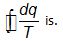JEE  >  Thermodynamics MCQ - 3 (Advanced)

# Thermodynamics MCQ - 3 (Advanced)

Test Description

## 21 Questions MCQ Test Chemistry for JEE Advanced | Thermodynamics MCQ - 3 (Advanced)

Thermodynamics MCQ - 3 (Advanced) for JEE 2022 is part of Chemistry for JEE Advanced preparation. The Thermodynamics MCQ - 3 (Advanced) questions and answers have been prepared according to the JEE exam syllabus.The Thermodynamics MCQ - 3 (Advanced) MCQs are made for JEE 2022 Exam. Find important definitions, questions, notes, meanings, examples, exercises, MCQs and online tests for Thermodynamics MCQ - 3 (Advanced) below.
 1 Crore+ students have signed up on EduRev. Have you?
Thermodynamics MCQ - 3 (Advanced) - Question 1

### One mole of an ideal diatomic gas(CV = 5 cal) was transformed from initial 25°C and 2 L to the state when temperature is 200°C and volume 10 L. Then for this process(R = 2 calories/moIN) (take calories as unit of energy and kelvin for temperature)

Detailed Solution for Thermodynamics MCQ - 3 (Advanced) - Question 1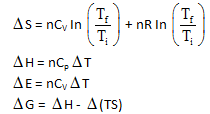Thermodynamics MCQ - 3 (Advanced) - Question 2

### Which of the following statements) is/are false?

Thermodynamics MCQ - 3 (Advanced) - Question 3

### Chemical reactions are invariably associated with transfer of energy miner  in form of heat or light. In the laboratory, heat changes in physical and chemical processes are measured with an instrument called calorimeter. Heat change in the process is calculated as q = ms Δ T                s = specific heat J/K-gm =C Δ T                          C = heat capacity J/K In Bomb calorimeter measurement are taking at constant volume. So heat of reaction at constant volume : q = ΔU (Internal energy change) Here heat is released by combustloin so sign of AU and heat should be negative. In water colarimeter measurement are taking at constant pressure. So, qp = Δ H qp = qv + P Δ V Δ H = Δ U + Δ ngRT Match the list-I and list-II and select the answer : List-I (Unbalanced reaction)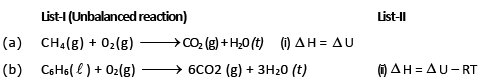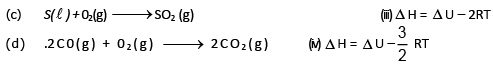Detailed Solution for Thermodynamics MCQ - 3 (Advanced) - Question 3

ΔH = ΔU + ΔngRT        for

(a)  Δng = - 2

(b)  Δ ng = - 3/2

(c)  Δ ng = 0

(d)  Δ ng = - 1

Thermodynamics MCQ - 3 (Advanced) - Question 4

Chemical reactions are invariably associated with transfer of energy miner  in form of heat or light. In the laboratory, heat changes in physical and chemical processes are measured with an instrument called calorimeter. Heat change in the process is calculated as

q = ms Δ T                s = specific heat J/K-gm

=C Δ T                          C = heat capacity J/K

In Bomb calorimeter measurement are taking at constant volume.
So heat of reaction at constant volume :

q = ΔU (Internal energy change)

Here heat is released by combustloin so sign of AU and heat should be negative. In water colarimeter measurement are taking at constant pressure.
So, qp = Δ H

qp = qv + P Δ V

Δ H = Δ U + Δ ngRT

Q.

What volume of AT should be used for the calorimeter experiment when the following graphical results are obtained ?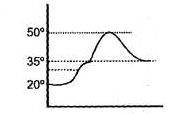Detailed Solution for Thermodynamics MCQ - 3 (Advanced) - Question 4

From combustion of fuel, the temp of calorimer raises and reaches to maximum value and then it decreases due to heat loss to the surrounding.

So,          ΔT = Tmax – Tinitial = 50 – 20 = 30°

Thermodynamics MCQ - 3 (Advanced) - Question 5

Chemical reactions are invariably associated with transfer of energy miner  in form of heat or light. In the laboratory, heat changes in physical and chemical processes are measured with an instrument called calorimeter. Heat change in the process is calculated as

q = ms Δ T                s = specific heat J/K-gm

=C Δ T                          C = heat capacity J/K

In Bomb calorimeter measurement are taking at constant volume.
So heat of reaction at constant volume :

q = ΔU (Internal energy change)

Here heat is released by combustloin so sign of AU and heat should be negative. In water colarimeter measurement are taking at constant pressure.
So, qp = Δ H

qp = qv + P Δ V

Δ H = Δ U + Δ ngRT

Q.

The heat capacity of a bomb calorimeter is 100 J/K. When 1 gm C7H16 (1) was burnt in this calorimeter, the temperature increase by 5°C. The value of Δ H in KJ/mole at 300 K temperature will be —

Detailed Solution for Thermodynamics MCQ - 3 (Advanced) - Question 5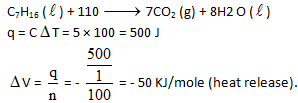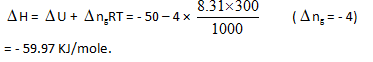Thermodynamics MCQ - 3 (Advanced) - Question 6

J.W. Gibbs and H.Von Helmoltz had given two equation and are known as Gibbs-Helmholtz equation. One equation can be expressed in terms of change in free energy (ΔG) and enthalpy (ΔHD) while other can be expressed in terms of change in internal energy (ΔE) and work function (ΔW)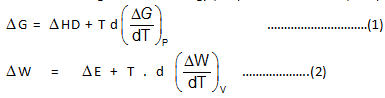Where T is temperature equation (1) is obtained at constant pressure while equation (B) is obtained at constant volume system. It is observed that for the reaction.

N2(g) + 3H2(g) → 2NH3(g)

Free energy change at 25°C is –33 kJ while at 35°C is —28 kJ which are at a constant pressure.

Q.

What would be the difference between enthalpy change at 25°C and 35°C for a given reaction :

Detailed Solution for Thermodynamics MCQ - 3 (Advanced) - Question 6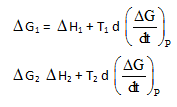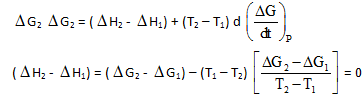Thermodynamics MCQ - 3 (Advanced) - Question 7

J.W. Gibbs and H.Von Helmoltz had given two equation and are known as Gibbs-Helmholtz equation. One equation can be expressed in terms of change in free energy (ΔG) and enthalpy (ΔHD) while other can be expressed in terms of change in internal energy (ΔE) and work function (ΔW)Where T is temperature equation (1) is obtained at constant pressure while equation (B) is obtained at constant volume system. It is observed that for the reaction.

N2(g) + 3H2(g) → 2NH3(g)

Free energy change at 25°C is –33 kJ while at 35°C is —28 kJ which are at a constant pressure.

Q.

What would be the free energy change at 30°C –

Detailed Solution for Thermodynamics MCQ - 3 (Advanced) - Question 7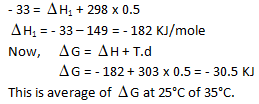Thermodynamics MCQ - 3 (Advanced) - Question 8

Internal energy change at 25°C is E1 while at 35°C is E2 then –

Detailed Solution for Thermodynamics MCQ - 3 (Advanced) - Question 8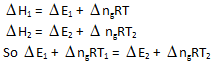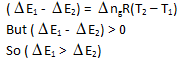Thermodynamics MCQ - 3 (Advanced) - Question 9

Internal Energy (E, also denoted by U) :

Every system having some quantity of matter is associated with a definite amount of energy, called internal energy

E = E Translational +ERotational + Evibrational + Ebounding+ …………

Δ E =  EFinal + Einitial

Δ E = qv , heat supplied to a gas at constant volume, since all the heat supplied goes to increase the internal energy of the gas .
It is an extensive property & a state function . It is exclusively a function of temperature.

If Δ T = 0 ; Δ E = 0 as well .
The internal energy of a certain substance is given by the following equation :

u = 3 pv + 84

where u is given in kJ/kg, p is in kPa, and v is in m3/kg A system composed of 3 kg of this substance expands from an initial pressure of 400 kPa and a volume of 0.2 m3 to a final pressure 100 kPa in a process in which pressure and volume are related by pv2 = constant.

Q.

If the expansion is quasi-static, then the value of Q is :

Detailed Solution for Thermodynamics MCQ - 3 (Advanced) - Question 9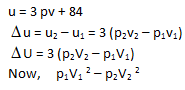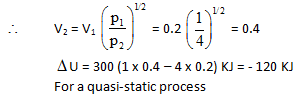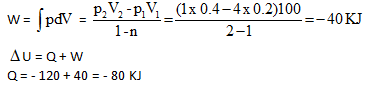Thermodynamics MCQ - 3 (Advanced) - Question 10

Internal Energy (E, also denoted by U) :

Every system having some quantity of matter is associated with a definite amount of energy, called internal energy

E = E Translational +ERotational + Evibrational + Ebounding+ …………

Δ E =  EFinal + Einitial

Δ E = qv , heat supplied to a gas at constant volume, since all the heat supplied goes to increase the internal energy of the gas .
It is an extensive property & a state function . It is exclusively a function of temperature.

If Δ T = 0 ; Δ E = 0 as well .
The internal energy of a certain substance is given by the following equation :

u = 3 pv + 84

where u is given in kJ/kg, p is in kPa, and v is in m3/kg A system composed of 3 kg of this substance expands from an initial pressure of 400 kPa and a volume of 0.2 m3 to a final pressure 100 kPa in a process in which pressure and volume are related by pv2 = constant.

Q.

In another process the same system expands according to the same pressure-volume relationship as in above question, and from the same initial state to the same final state as in above question, but the heat transfer in this case is 30 kJ. Then the work transfer for this process is :

Detailed Solution for Thermodynamics MCQ - 3 (Advanced) - Question 10

Since the end states are the same, ΔU would remain the same as in (a).

W = ΔU – Q

W = - 120 – 30 = - 150 KJ

Thermodynamics MCQ - 3 (Advanced) - Question 11

The enthalpy in the process
HCI + nH2O → HCI  in n moles of H20

where n is the number of moles of water, is called the integral heat of solution. When n is large enough that continued addition of water does not increase the heat of solution, one simply writes

HCI +aq →HCI (aq)

The enthalpy for this process is the limiting value for the integral heat of solution. The enthalpy for the process

HCI  in  n  moles of H2O + mH2O → HCI in (n + m) moles of H2O

is called the integral heat of dilution. These quantities are indicated in figure. Another quantity of interest is the differential heat of solution, defined as the slope of the enthalpy curve. The heats of solution depend on the composition of the solution as shown in the figure.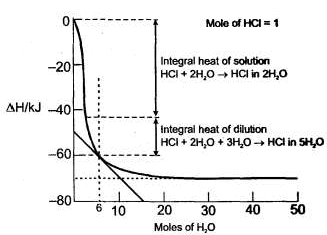Q.

Integral heat of solution for the following step is :

HCI + 5H20→ HCI (5H20)

Thermodynamics MCQ - 3 (Advanced) - Question 12

The enthalpy in the process
HCI + nH2O → HCI  in n moles of H20

where n is the number of moles of water, is called the integral heat of solution. When n is large enough that continued addition of water does not increase the heat of solution, one simply writes

HCI +aq →HCI (aq)

The enthalpy for this process is the limiting value for the integral heat of solution. The enthalpy for the process

HCI  in  n  moles of H2O + mH2O → HCI in (n + m) moles of H2O

is called the integral heat of dilution. These quantities are indicated in figure. Another quantity of interest is the differential heat of solution, defined as the slope of the enthalpy curve. The heats of solution depend on the composition of the solution as shown in the figure.Q.

What is the enthalpy change for the reaction HCI (aq) →HCl(aq)

Thermodynamics MCQ - 3 (Advanced) - Question 13

The enthalpy in the process
HCI + nH2O → HCI  in n moles of H20

where n is the number of moles of water, is called the integral heat of solution. When n is large enough that continued addition of water does not increase the heat of solution, one simply writes

HCI +aq →HCI (aq)

The enthalpy for this process is the limiting value for the integral heat of solution. The enthalpy for the process

HCI  in  n  moles of H2O + mH2O → HCI in (n + m) moles of H2O

is called the integral heat of dilution. These quantities are indicated in figure. Another quantity of interest is the differential heat of solution, defined as the slope of the enthalpy curve. The heats of solution depend on the composition of the solution as shown in the figure.Q.

Approximate value of differential heat of that solution in which 1 mole of CI is dissolved in 6 mole of water is:

Thermodynamics MCQ - 3 (Advanced) - Question 14

A gaseous sample is generally allowed to do only expansion/compression type of work against its surroundings. The work done in case of an irreversible expansion (in the intermediate stages, of expansion/compression the states of gases are not defined). The work done can be calculated using

dW = –NextdV

while in case of reversible process the work done can be calculated using dw = –PdV where P is pressure of gas at some intermediate stages. Like for an isothermal reversible process.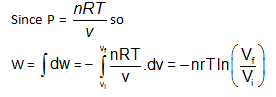Since d w  = – P d V so magnitude of worked one can also be calculated by, calculating the area under the PV curve of the reversible process in PV diagram.

Q.

An ideal gaseous sample at initial state i (P0V0T0) is allowed to expand to volume 2V0 using two different process; in the first process the equation of process is PV2= K1 and in second process the equation of the process is PV = K2 .Then

Detailed Solution for Thermodynamics MCQ - 3 (Advanced) - Question 14

Work done in isothermal process will be more than PV2 = const process whatever the value of K1 and K2 is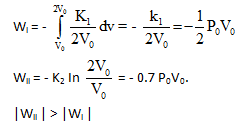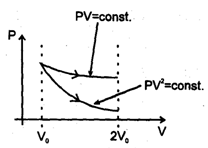Thermodynamics MCQ - 3 (Advanced) - Question 15

A gaseous sample is generally allowed to do only expansion/compression type of work against its surroundings. The work done in case of an irreversible expansion (in the intermediate stages, of expansion/compression the states of gases are not defined). The work done can be calculated using

dW = –NextdV

while in case of reversible process the work done can be calculated using dw = –PdV where P is pressure of gas at some intermediate stages. Like for an isothermal reversible process.Since d w  = – P d V so magnitude of worked one can also be calculated by, calculating the area under the PV curve of the reversible process in PV diagram.

Q.

There are two samples of same gas initially under similar initial state. Gases of both the samples are expanded. Ist sample using reversible isothermal process and IInd sample using reversible adiabatic process till final pressures of both the samples becomes half of initial pressure, then

Detailed Solution for Thermodynamics MCQ - 3 (Advanced) - Question 15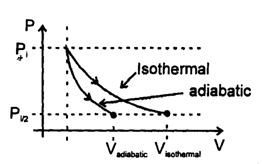Thermodynamics MCQ - 3 (Advanced) - Question 16

A gaseous sample is generally allowed to do only expansion/compression type of work against its surroundings. The work done in case of an irreversible expansion (in the intermediate stages, of expansion/compression the states of gases are not defined). The work done can be calculated using

dW = –NextdV

while in case of reversible process the work done can be calculated using dw = –PdV where P is pressure of gas at some intermediate stages. Like for an isothermal reversible process.Since d w  = – P d V so magnitude of worked one can also be calculated by, calculating the area under the PV curve of the reversible process in PV diagram.

Q.

In the above problem

Detailed Solution for Thermodynamics MCQ - 3 (Advanced) - Question 16

Magnitude of work done in isothermal expansion process is more than adiabatic expansion process.

Thermodynamics MCQ - 3 (Advanced) - Question 17

A gaseous sample is generally allowed to do only expansion/compression type of work against its surroundings. The work done in case of an irreversible expansion (in the intermediate stages, of expansion/compression the states of gases are not defined). The work done can be calculated using

dW = –NextdV

while in case of reversible process the work done can be calculated using dw = –PdV where P is pressure of gas at some intermediate stages. Like for an isothermal reversible process.Since d w  = – P d V so magnitude of worked one can also be calculated by, calculating the area under the PV curve of the reversible process in PV diagram.

Q.

If four identical samples of an ideal gas initially at similar state (P0, Vo, T0) are allowed to expand to double their volumes by four different processes..I : by isothermal irreversible process II :  by reversible process having equation P2V = constant III : by reversible adiabatic process IV: by irreversible adiabatic expansion against constant external pressure.Then in the graph shown the final state is represented by four different points then, the correct match can be :

Detailed Solution for Thermodynamics MCQ - 3 (Advanced) - Question 17

Work done is least incase of reversible adiabatic process. Work done will be greatest in the process P2V = Constant

Thermodynamics MCQ - 3 (Advanced) - Question 18

A gaseous sample is generally allowed to do only expansion/compression type of work against its surroundings. The work done in case of an irreversible expansion (in the intermediate stages, of expansion/compression the states of gases are not defined). The work done can be calculated using

dW = –NextdV

while in case of reversible process the work done can be calculated using dw = –PdV where P is pressure of gas at some intermediate stages. Like for an isothermal reversible process.Since d w  = – P d V so magnitude of worked one can also be calculated by, calculating the area under the PV curve of the reversible process in PV diagram.

Q.

Two samples (initially under same states) of an ideal gas are first allowed to expand to double their volume using irreversible isothermal expansion against constant external pressure, then samples are returned back to their original volume first by reversible adiabatic process and second by reversible process having equation PV2 = constant then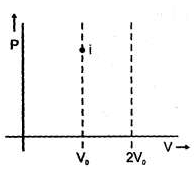Detailed Solution for Thermodynamics MCQ - 3 (Advanced) - Question 18

Processes may be represented as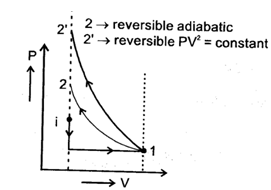If initial temperature is same then temperature in 2nd case is more.

Thermodynamics MCQ - 3 (Advanced) - Question 19

Spontaneity of any process can be predicted with the help of D Stotal. But this requires calculation of changes in system as will as surroundings. If some criteria (depending upon the system only) can be developed for checking spontaneity under specific conditions, then that would be a more useful parameter. The criteria can be derived from Clausius inequality.
T dS≥q, > sign for rev. process

= sign for rev. process

or T dS > dB – W for an irr. Process

or T dSsys > dUsys + p dV [consider no non-PV work]

If U and V are constant, T dSsys>  0

for spontaneous process

If V and T are constant

T dS > dU

or dB — T dS < 0

As temperature is constant, dU – d(TS) < 0 or d (U –TS) <  0

Another state function A(Helmholtz's function) = B – TS A decrease in Helmholtz function (A) under constant volume and temperature is the criteria of spontaneity of a process.

Q.

For a spontaneous process, if entropy and volume are constant, the internal energy of system must

Thermodynamics MCQ - 3 (Advanced) - Question 20

Spontaneity of any process can be predicted with the help of D Stotal. But this requires calculation of changes in system as will as surroundings. If some criteria (depending upon the system only) can be developed for checking spontaneity under specific conditions, then that would be a more useful parameter. The criteria can be derived from Clausius inequality.
T dS≥q, > sign for rev. process

= sign for rev. process

or T dS > dB – W for an irr. Process

or T dSsys > dUsys + p dV [consider no non-PV work]

If U and V are constant, T dSsys>  0

for spontaneous process

If V and T are constant

T dS > dU

or dB — T dS < 0

As temperature is constant, dU – d(TS) < 0 or d (U –TS) <  0

Another state function A(Helmholtz's function) = B – TS A decrease in Helmholtz function (A) under constant volume and temperature is the criteria of spontaneity of a process.

Q.

If a state function G is defined such as O = H – TS, then dO must decrease for a spontaneous process (involving only PV work) occuring at

Thermodynamics MCQ - 3 (Advanced) - Question 21

Spontaneity of any process can be predicted with the help of D Stotal. But this requires calculation of changes in system as will as surroundings. If some criteria (depending upon the system only) can be developed for checking spontaneity under specific conditions, then that would be a more useful parameter. The criteria can be derived from Clausius inequality.
T dS≥q, > sign for rev. process

= sign for rev. process

or T dS > dB – W for an irr. Process

or T dSsys > dUsys + p dV [consider no non-PV work]

If U and V are constant, T dSsys>  0

for spontaneous process

If V and T are constant

T dS > dU

or dB — T dS < 0

As temperature is constant, dU – d(TS) < 0 or d (U –TS) <  0

Another state function A(Helmholtz's function) = B – TS A decrease in Helmholtz function (A) under constant volume and temperature is the criteria of spontaneity of a process.

Q.

For an irreversible cyclic process